# Processing algorithms in the QGis 2.8 Graphic Modeler

Adding Algorithms in the Graphic Modeler

The algorithms are added in   the graphical modeler in the same way as the input data. You simply select the tab   algorithms   instead of the tab   Inputs, to see the display corresponding to the panel   Toolbox of treatments .Once you have found the algorithm you want to add, double-click on it, or drag it to the Modeler graphic window. In order to find the algorithm, you just research in the Processing Toolbox, typing the name in the search box at the top of the algorithm tab.

In fact, the tab   algorithms   is the opened window of the toolbox in the modeler. The display of this box has two parameters: Advanced and Simplified.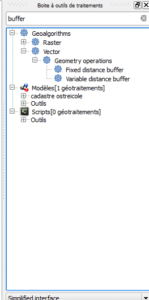Display with simplified interface

The parameter of the interface that is currently used in the processing toolbox determines how the algorithms will be organized in the graphical modeler. This parameter cannot be changed in the graphical modeler. To change the setting, the graphical modeler must be stopped, the interface parameter must be changed in the toolbox and the graphical modeler must be restarted. Apart from that, finding algorithms follows the same approach as in the treatment toolbox: type the name or a part of the name of the desired treatment and you will have the list of available treatments.

In the algorithms tab, there is a special category called   Modeler specific tools. The three tools – Calculator, Raster Layers Limits, and Vector Layers Limits – do not appear in the toolbox. These are tools that make sense only when used as part of the graphical modeler.

The calculator tool is   the most commonly used of the three tools. It allows you to perform arithmetic calculations on the digital outputs of other algorithms, and to insert them as input to other treatments of the same model.

When you add the calculator to the graphics window, the info window   lists the​​available digital values ​​ within the model. The window below is used to enter a calculation formula by using the letters assigned to each numerical value as variables. For example, the formula entered   in the following figure   displays the only digital variable of our current model (the buffer distance) and calculates twice that distance (a * 2).This output can be used as input to another algorithm of this model.

When you add an algorithm to the graphical window of the modeler, the parameter window of the algorithm opens. The dialog box will be very similar to the one you would have if you had launched the tool directly from the processing box: you will have the input data, the output data and the tool parameters.However, there are some important differences since the graphical modeler is an autonomous universe of data entries. The differences are the following:
• In the list of   input layers   you will be limited to those that have been added to the template.• The field   Exit   can be left empty if it is an intermediate result that will be used as input for another algorithm. If the output is a layer that needs to be saved, enter the name of that layer in the text box. When you name an output layer, you do not need to provide an output file name. This will be done when the tool is run. Instead, just enter the name of the layer (for example, buffer installations).
It is important to understand how the output data of an algorithm works:

• If they are used as intermediate data for the following algorithms, then:   you leave the name empty
• If they are used by the following algorithms, you want to keep the layer produced, then:   you give a name.

This name is not the name of the output file, it is the label that will be displayed in the model execution window when you execute it. Remember that in the modeler, during the model creation the data is not used. You use labels that will be replaced by the actual data only when you run the model.Digital values ​​or string parameters can be entered directly as numbers or strings, but they can also be selected from the number or string type inputs.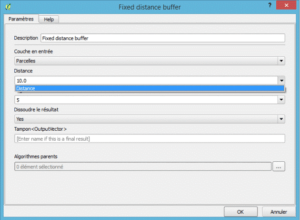• Fields in an attribute table (or other independent table) can be specified by typing the field name or using the Table field entry.These fields will be chosen when the model is run.
• Parent Algorithms is an additional parameter available only with tools running through the Graphical Modeler.It allows you to define the order of execution of the algorithms.Defining an algorithm as a parent forces the graphical modeler to execute the algorithm before, actually, running it. Depending on the complexity of the model, you can have multiple branches that join or separate. The execution order of the algorithms will be defined by QGis when run, but there is no guarantee that one algorithm will be executed before another, EXCEPT if you use the option   Parent Algorithm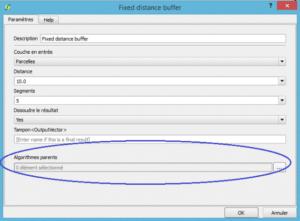On the other hand, if you have a linear model, without branches, when you define the output of an algorithm as input for the next one, you automatically define the first algorithm as the parent of the second one.

In our example, we will perform as a first algorithm the calculation of a buffer zone around oyster facilities.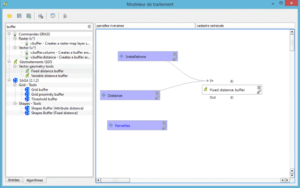Note that instead of setting an explicit buffer distance, the buffer distance entry is used. Also note that no output is named since this output will be considered an intermediate dataset.

To complete our model, we will add the Clip tool in the model.We will use the following parameters:
• Input layer:   plots
• Cutting layer: “Buffer” of the “fixed distance buffer” algorithm
• Output layer (OutputVector): Riparian plotsThe final template looks like the following screenshot.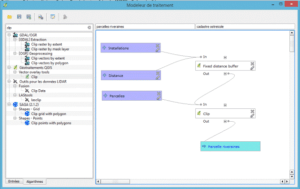The connecting lines show how the elements are linked to each other in the workflow. Inputs, outputs and algorithms are represented by boxes of different colours. The boxes of the algorithms also contain, on the left, an icon representing the library source. In our example, both algorithms are QGIS algorithms and have the icon Q in the box of the item.

The template is now ready to run.